# LINQ OrderBy Method in C#

## LINQ OrderBy Method in C# with Examples

In this article, I will discuss the LINQ OrderBy Method in C# with Examples. Please read our previous article discussing the basics of LINQ Ordering Operators. As part of this article, we are going to discuss the following pointers, which are related to the LINQ OrderBy method.

1. What is the LINQ OrderBy Method?
2. Example of LINQ OrderBy Method using both Method and Query Syntax.
3. How to use the LINQ OrderBy Method with Complex Type in C#?
4. How do you use the OrderBy method along with the Filtering method?
5. How to Create and Use Own Comparer with LINQ OrderBy Method in C#?
6. When to Use the LINQ OrderBy Method?
##### What is the Linq OrderBy Method?

The LINQ OrderBy Method in C# is used for sorting elements in a collection based on specified key(s). The LINQ OrderBy method in C# is used to sort the data in Ascending Order. It is a part of the System.Linq namespace can be used with arrays, lists, or any other type that implements the IEnumerable<T> interface.

This method is not going to change the data. Rather, it is just going to change the order of the data. You can use the OrderBy method on any data type, i.e., you can use character, string, decimal, integer, etc. There are two overloaded versions of this OrderBy Method available inside LINQ. They are as follows.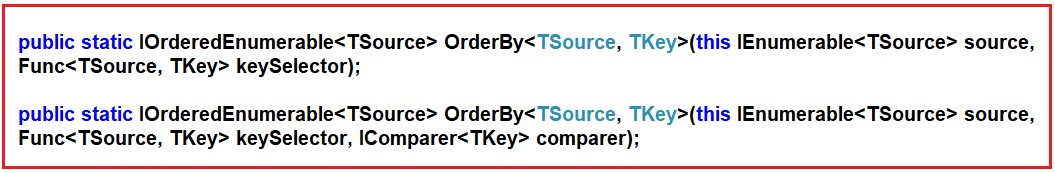The only difference between these two overloaded versions is that the second version takes the IComparer parameter, which compares the keys when creating our custom compare. Let us understand using the above two LINQ OrderBy methods in C# using Query Syntax and Method Syntax.

##### LINQ OrderBy Method with Value Data Type in C#

Let us see an example of how the LINQ OrderBy Method works with Value Data Type using C#. In the below example, we have a collection of integer data. Then, we sort the data in ascending order using the LINQ OrderBy method using both Method and Query Syntax.

```using System;
using System.Collections.Generic;
using System.Linq;
namespace LINQDemo
{
class Program
{
static void Main(string[] args)
{
List<int> intList = new List<int>() { 10, 45, 35, 29, 100, 69, 58, 50 };

Console.WriteLine("Before Sorting : ");
foreach (var item in intList)
{
Console.Write(item + " ");
}

//Sorting the data in Ascending Order
//Using Method Syntax
var MS = intList.OrderBy(num => num);

//Using Query Syntax
var QS = (from num in intList
orderby num
select num).ToList();

Console.WriteLine();
Console.WriteLine("After Sorting : ");
foreach (var item in QS)
{
Console.Write(item + " ");
}

}
}
}```

Now, run the application, and you will get the following output.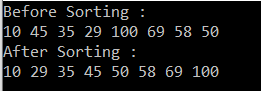##### OrderBy Method Key Characteristics:
• The OrderBy method sorts elements in ascending order based on a specified key.
• By default, sorting is case-sensitive and in ascending order. To sort in descending order, you can use the OrderByDescending method.
• The original sequence remains unchanged; OrderBy returns a new ordered sequence.
• You can use the OrderBy method with various data types and custom classes if the collection implements IEnumerable<T>.
• They are deferred execution methods, meaning the sorting doesn’t happen until the sorted collection is iterated.
##### LINQ OrderBy Method with String Data Type in C#

Let us see an example of how the LINQ OrderBy Method works with String Data Type using C#. In the below example, we have a collection of string names. We then sort the data in ascending order using the LINQ OrderBy method with both Method and Query syntax.

```using System;
using System.Collections.Generic;
using System.Linq;
namespace LINQDemo
{
class Program
{
static void Main(string[] args)
{
List<string> stringList = new List<string>() { "Preety", "Tiwary", "Agrawal", "Priyanka", "Dewangan",
"Hina","Kumar","Manoj", "Rout", "James"};

//Using Method Syntax
var MS = stringList.OrderBy(name => name);

//Using Query Syntax
var QS = (from name in stringList
orderby name ascending
select name).ToList();

foreach (var item in MS)
{
Console.WriteLine(item + " ");
}

}
}
}```

When you run the above code, you will get the following output.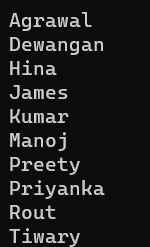Note: In the Query Syntax, the ascending operator is optional while sorting the data in ascending order. That means if we are not specifying anything, then by default, it is ascending. So, the following two statements are the same.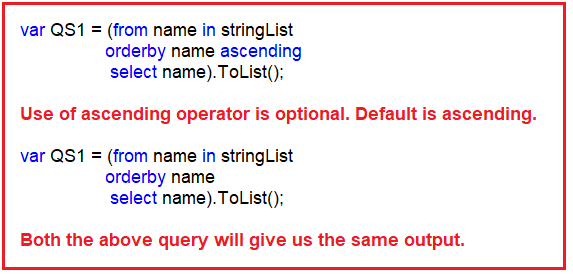##### LINQ OrderBy Method with Complex Data Type in C#:

Let us see how the LINQ OrderBy Method works with Complex Data Types in C# with some Examples. We are going to work with the following Student class. So, create a class file named Student.cs and copy and paste the following code. As you can see, we created the Student class with four properties: ID, FirstName, LastName, and Brach. We then created one method, i.e., GetAllStudents(), to return a list of students.

```using System.Collections.Generic;
namespace LINQDemo
{
public class Student
{
public int ID { get; set; }
public string FirstName { get; set; }
public string LastName { get; set; }
public string Branch { get; set; }

public static List<Student> GetAllStudents()
{
List<Student> listStudents = new List<Student>()
{
new Student{ID= 101,FirstName = "Preety",LastName = "Tiwary",Branch = "CSE"},
new Student{ID= 102,FirstName = "Preety",LastName = "Agrawal",Branch = "ETC"},
new Student{ID= 103,FirstName = "Priyanka",LastName = "Dewangan",Branch = "ETC"},
new Student{ID= 104,FirstName = "Hina",LastName = "Sharma",Branch = "ETC"},
new Student{ID= 105,FirstName = "Anugrag",LastName = "Mohanty",Branch = "CSE"},
new Student{ID= 106,FirstName = "Anurag",LastName = "Sharma",Branch = "CSE"},
new Student{ID= 107,FirstName = "Pranaya",LastName = "Kumar",Branch = "CSE"},
new Student{ID= 108,FirstName = "Manoj",LastName = "Kumar",Branch = "ETC"},
new Student{ID= 109,FirstName = "Pranaya",LastName = "Rout",Branch = "ETC"},
new Student{ID= 110,FirstName = "Saurav",LastName = "Rout",Branch = "CSE"}
};

return listStudents;
}
}
}```

Now, we want to sort the student data based on the Branch of the Student in ascending order. To do so, modify the Main method of the Program class as follows. In the below example, we are Sorting the data in ascending order based on the student branch using LINQ OrderBy Method with both Method and Query Syntax.

```using System.Linq;
using System;
namespace LINQDemo
{
class Program
{
static void Main(string[] args)
{
//Method Syntax
var MS = Student.GetAllStudents().OrderBy(x => x.Branch).ToList();

//Query Syntax
var QS = (from std in Student.GetAllStudents()
orderby std.Branch
select std);

foreach (var student in MS)
{
Console.WriteLine(" Branch: " + student.Branch + ", Name :" + student.FirstName + " " + student.LastName );
}

}
}
}```

When you run the above code, you will get the following output.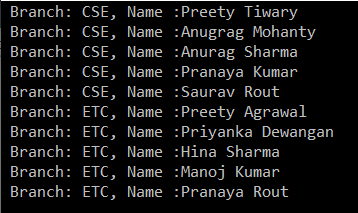##### Sorting with Filtering using LINQ

Now we need to fetch only the CSE branch students, and then we need to sort the data based on the FirstName in Ascending order. The most important point you need to remember is to use the Where method before the OrderBy method. This is because it will filter and sort the filtered results, improving the application’s performance. The following example uses LINQ OrderBy and Where Method with Query and Method Syntax.

```using System;
using System.Collections.Generic;
using System.Linq;
namespace LINQDemo
{
class Program
{
static void Main(string[] args)
{
//Method Syntax
var MS = Student.GetAllStudents()
.Where(std => std.Branch.ToUpper() == "CSE")
.OrderBy(x => x.FirstName).ToList();

//Query Syntax
var QS = (from std in Student.GetAllStudents()
where std.Branch.ToUpper() == "CSE"
orderby std.FirstName
select std);

foreach (var student in QS)
{
Console.WriteLine(" Branch: " + student.Branch + ", Name :" + student.FirstName + " " + student.LastName );
}

}
}
}
```

When you run the above code, you will get the following output as expected.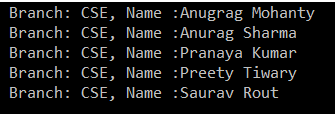##### How to Create and Use Own Comparer with LINQ OrderBy Method in C#?

Creating and using our Comparer with the LINQ OrderBy Method is also possible. We need to use the Second Overloaded version of the OrderBy Method, which takes the IComparer parameter. For a better understanding, please have a look at the below example.

```using System;
using System.Collections.Generic;
using System.Linq;
namespace LINQDemo
{
public class CaseInsensitiveComparer : IComparer<string>
{
public int Compare(string x, string y)
{
return string.Compare(x, y, true);
}
}

public class MainClass
{
public static void Main()
{
CaseInsensitiveComparer caseInsensitiveComparer = new CaseInsensitiveComparer();
string[] Alphabets = { "a", "b", "c", "A", "B", "C"};
var SortedAlphabets = Alphabets.OrderBy(aplhabet => aplhabet, caseInsensitiveComparer);
foreach (var item in SortedAlphabets)
{
Console.Write(item + " ");
}
}
}
}```

Output: a A b B c C

##### When to Use the LINQ OrderBy Method in C#?

Here are some common use cases for employing the OrderBy method:

• Sorting Data for Display: When presenting data to users, sorting can improve readability and usability. For instance, sorting a list of names alphabetically, products by price, or files by date.
• Data Analysis: In data processing or analysis tasks, sorting data can be an initial step to organize it for pattern detection, statistical analysis, or simplifying complex operations.
• Improving Query Efficiency: In certain cases, sorting data can make subsequent queries more efficient, especially when combined with operations like First or FirstOrDefault, where you might be interested in the top item according to some criteria.
• Ordering Results of a LINQ Query: Often, OrderBy is used at the end of a LINQ query chain to ensure that the results are returned in a specified order.
• Database Queries: When using LINQ with databases (like LINQ to SQL or Entity Framework), OrderBy translates to an SQL ORDER BY clause, providing a way to sort data at the database level before it’s loaded into memory.
• Custom Sorting Logic: OrderBy can be used with a custom comparer or a lambda expression, offering flexibility to define complex sorting logic beyond simple property-based sorting.

In the next article, I will discuss sorting the data in descending order using the LINQ OrderByDescending Method in C# with Examples. In this article, I explain the need and use of the LINQ OrderBy Method in C# with Examples. I hope you enjoy this OrderBy Method in C# article.